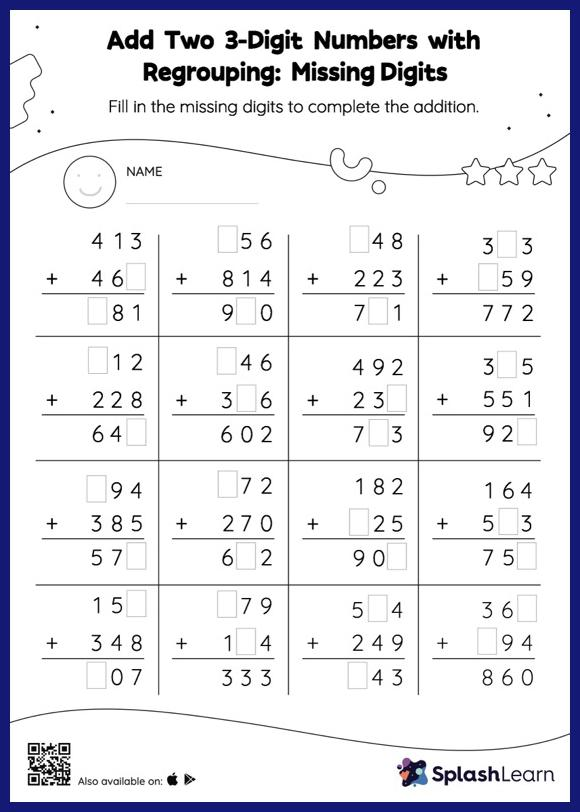# Add Two 3-Digit Numbers with Regrouping: Missing Digits Worksheet

Home > Add Two 3-Digit Numbers with Regrouping: Missing DigitsAdd two 3-digit numbers with regrouping worksheet helps students develop proficiency with addition. To find the missing number on this worksheet, students can utilize the count-on strategy or the connection between addition and subtraction. In add two 3-digit numbers with regrouping worksheet, they must additionally regroup numbers to reach the solution. This worksheet is about practicing the column method in which numbers are written one on top of another. In this method, students use their place value understanding to solve the problems.